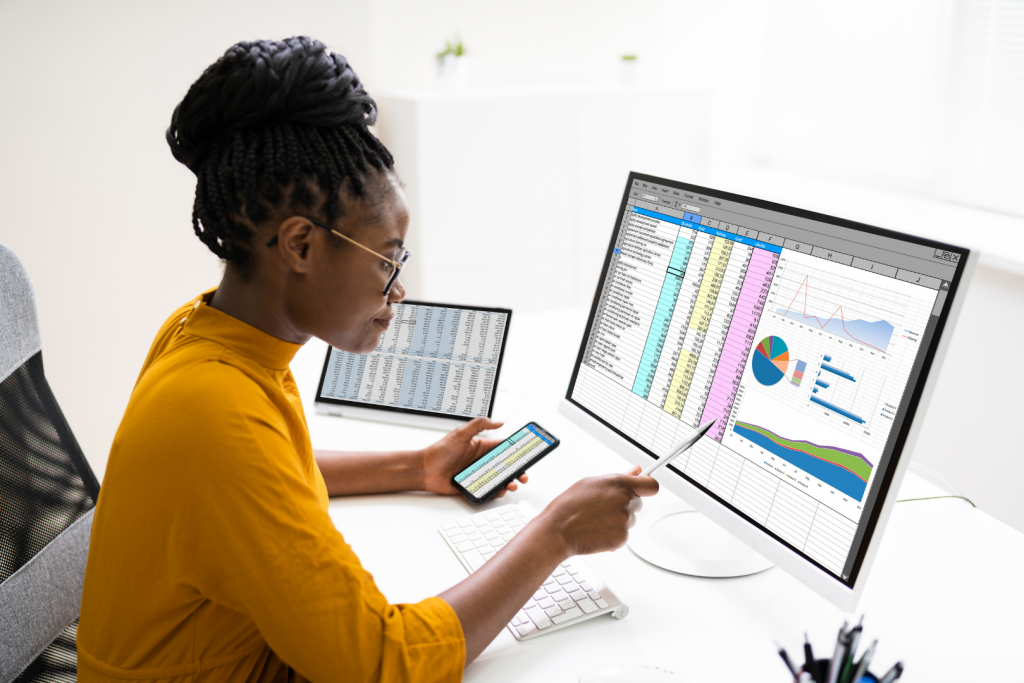## Statistical Analysis and Review Services

Let our expert statisticians save you dozens of hours and provide insights to help strengthen your research. Since 1994, we have helped researchers with over 3,500 stats projects. We guarantee you will be happy and provide a full money back guarantee.## Statistical Packages

Choose from the following statistical analysis packages based on your needs.

### Level I Statistical Analysis

\$ 249
• Organizing and preparing your data for analysis
• Conducting a Normality test to identify trends and outliers
• Descriptive statistics and summary, and frequency tables
• Optimizing visual presentation with appropriate graphs
• Performing basic analysis using Chi-Square tests and T-tests
• Performing Multiple Regression with up to 5 models with up to 10 variables in each model.
• Summary highlights of the results
• Appropriate graphs and charts

### Level II Statistical Analysis

\$ 399
• Repeat the analysis to corroborate the results and confirm reproducibility
• Get useful feedback on statistical methods, analysis, and results, with advice for corrective action
• Multiple Regression, ANOVA, ANACOVA for up to 10 models with up to 20 variables
• Logistic Regression, discrete analysis for up to 10 models with up to 20 variables
• Structural Equation Modeling with up to 30 questions and 300 respondents
• Factor Analysis with up to 30 questions and 300 respondents
• Create up to 5 Predictive models
• Intermediate Econometric models
• Intermediate Financial models (e.g., GARCH, MA, ARIMA)
• Data Cleansing
• Summary highlights of the results
• Appropriate graphs and charts

### Level III Statstical Analysis

\$ 699
• Draw crucial inferences from the results
• Present relevant results in tables/graphs
• Detect potential limitations and weaknesses
• Multiple Regression, ANOVA, ANACOVA for up to 15 models with up to 150 variables
• Logistic Regression, discrete analysis for up to 10 models with up to 20 variables
• Structural Equation Modeling with up to 50 questions and 500 respondents
• Factor Analysis with up to 50 questions and 500 respondents
• Create up to 30 advanced Predictive models